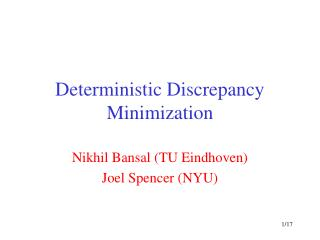# Deterministic Discrepancy Minimization - PowerPoint PPT PresentationDownload PresentationDeterministic Discrepancy Minimization

Deterministic Discrepancy MinimizationDownload Presentation## Deterministic Discrepancy Minimization

- - - - - - - - - - - - - - - - - - - - - - - - - - - E N D - - - - - - - - - - - - - - - - - - - - - - - - - - -
##### Presentation Transcript

1. Deterministic Discrepancy Minimization Nikhil Bansal (TU Eindhoven) Joel Spencer (NYU)

2. S3 S4 S1 S2 Combinatorial Discrepancy Universe: U= [1,…,n] Subsets: S1,S2,…,Sm Problem: Color elements red/blue so each subset is colored as evenly as possible. CS: Computational Geometry, Comb. Optimization, Monte-Carlo simulation, Machine learning, Complexity, Pseudo-Randomness, … Math: Dynamical Systems, Combinatorics, Mathematical Finance, Number Theory, Ramsey Theory, Algebra, Measure Theory, …

3. General Set System Universe: U= [1,…,n] Subsets: S1,S2,…,Sm Find : [n] ! {-1,+1} to Minimize |(S)|1 = maxS | i 2 S(i) | For simplicity consider m=n henceforth.

4. Simple Algorithm Random: Color each element i independently as x(i) = +1 or -1 with probability ½ each. Thm: Discrepancy = O (n log n)1/2 Pf: For each set, expect O(n1/2) discrepancy Standard tail bounds: Pr[ | i 2 S x(i) | ¸c n1/2 ] ¼e-c2 Union bound + Choose c ¼ (log n)1/2 Analysis tight: Random actually incurs ((n log n)1/2).

5. Better Colorings Exist! [Spencer 85]: (Six standard deviations suffice) Always exists coloring with discrepancy ·6n1/2 Tight: Cannot beat 0.5 n1/2 (Hadamard Matrix, “orthogonal” sets) Inherently non-constructive proof (pigeonhole principle on exponentially large universe) Challenge: Can we find it algorithmically ? (Certain algorithms do not work) Conjecture[Alon-Spencer]: May not be possible.

6. Algorithmic Results [Bansal 10]: Efficient (randomized) algorithm for Spencer’s result. Technique: SDPs (new rounding idea) Use several SDPs over time (guided by the non-constructive method). General: Geometric problems, Beck Fiala setting, k-permutation problem, pseudo-approximation of discrepancy, … Thm: Deterministic Algorithm for Spencer’s (and other) results.

7. This Talk Goal: Round to -1 or 1 Minimize error for each row A Chernoff: Error = Spencer: Error =

8. Derandomizing Chernoff(Pessimistic estimators, exp. moments, hyp. cosine rule, …)

9. The Problem Such approaches cannot get rid of (Chooser-Pusher Games: Where each column rounded in an online manner) Algorithm of Bansal uses a more global approach

10. start finish Algorithm (at high level) Each dimension: A variable Each vertex: A rounding Cube: {-1,1}n Algorithm: At step t, update Fix variable if reaches -1 or 1. g: random is random Gaussian in Each distributed as a Gaussian But the ’s are correlated.

11. SDP relaxations SDPs(LP on ) “is small” 8 j |vi|2 = 1 Intended soln. vi = (+1,0,…,0) or (-1,0,…,0). Spencer’s result (entropy method) guarantees feasibility. Key point of Gaussian rounding: Say if Then

12. start finish Analysis (at high level) Each dimension: An Element Each vertex: A Coloring Cube: {-1,1}n Analysis: Progress: Few steps to reach a vertex (walk has high variance) Low Discrepancy: For each equation, discrepancy random walk has low variance

13. Making it Deterministic Need to find an update that • Makes Progress • Adds low discrepancy to equations. Recall, for Chernoff: Round one variable at a time (Progress) Whether -1 or +1, guided by the potential. (Low Discrepancy)

14. Tracking the properties i) For low discrepancy. Define suitable potential and bound its increase (as in Chernoff, but refined) ii) For Progress Potential Energy shouldgo up sufficiently Conflicting goals (hold in expectation) No reason why such an update should even exist.

15. Our fix Add extra constraints to SDP to force a good update to exist. Orthogonalitytrick: Say currently at Add SDP constraint Ensures that update orthogonal to x. The length (potential) always increases! Analogous constraint for low discrepancy potential (bounds increase by right amount) x(t-1) x(t): New position origin

16. Trouble Why should this SDP remainfeasible? In Bansal’s (randomized) algorithm SDP was feasible due to Spencer’s existential result. Key point: New constraint isof similar type i.e.is small) Entropy method: new SDP is still feasible. Finish off: Use k-wise vectors instead of Gaussian

17. Concluding Remarks Idea: Add new constraints to force a deterministic choice to exist. Works more generally for other discrepancy problems. Can potentially have other applications. Thank You!

18. Techniques Entropy Method Spencer’s Result SDPs Bansal’s Result New “orthogonality” idea (based on entropy) + K-wise independence, pessimistic estimators, … This Result